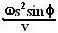## Friday, December 14, 2007

### Irodov Problem 1.111The earth is a rotating sphere (well almost). Suppose that its radius is R
and it is rotating at an angular speed ofand so are all things on it - the person, the gun, the bullet in it and the target.

The bullets's motion
The person stands at a longitude ofand hence his distance from the Earth's axis of rotation is. Because of the Earth's rotation about its axis the person and hence the gun and the bullet have a tangential velocity. After the bullet is shot it will continue to have this tangential velocity. Further, it will also be acted upon by gravity that will pull it radially inwards towards the ground. This is indicated in the figure.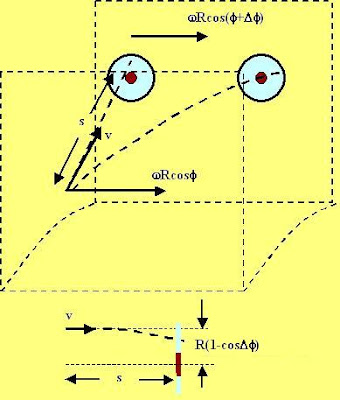The target's motion
The target that is s units away from the person further along the north. Suppose that the latitude here is. This means that,The target is thus at a distance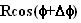from the axis of rotation. This means that from the point of view of the shooter, the target is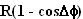below his horizontal level as shown in the figure. Also the tangential velocity of the target as it rotates with the earth iswhich is slightly less than the tangential velocity of the bullet. This is also depicted in the figure.

The time taken by the bullet to reach the target is s/v, and during this time it will fall a distance ofbelow the horizontal. The target is moving a bit slower tangentially then the bullet by a valueand so the bullet will move farther than the target tangentially.

Vertical Offset
Since 1km is much much smaller than the earth's radius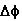will be very very small. The vertical offset will thus be,Horizontal offset
The horizontal offset is due to the difference in tangential velocities of the target and bullet given by,Also in the problemthis means that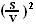will be negligibly small and we can neglect it. Given this the only displacement becomes